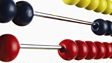A number and a quarter of that number add up to fifteen. What is the number? To work out the answer the presenter uses a tile split into four equal parts. Using simple addition, division, multiplication and a degree of logic, he shows how it is possible to work out the answer.This clip is from:
The Maths Channel, Fractions